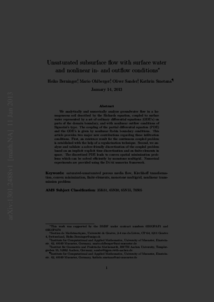Repository: Freie Universität Berlin, Math Department

# Unsaturated subsurface flow with surface water and nonlinear in- and outflow conditions

Berninger, H. and Ohlberger, M. and Sander, O. and Smetana, K. (2014) Unsaturated subsurface flow with surface water and nonlinear in- and outflow conditions. Mathematical Models and Methods in Applied Sciences, 24 (5). pp. 901-936. ISSN 1793-6314Preview

1MB

Official URL: https://dx.doi.org/10.1142/S0218202513500711

## Abstract

We analytically and numerically analyze groundwater flow in a homogeneous soil described by the Richards equation, coupled to surface water represented by a set of ordinary differential equations (ODE's) on parts of the domain boundary, and with nonlinear outflow conditions of Signorini's type. The coupling of the partial differential equation (PDE) and the ODE's is given by nonlinear Robin boundary conditions. This article provides two major new contributions regarding these infiltration conditions. First, an existence result for the continuous coupled problem is established with the help of a regularization technique. Second, we analyze and validate a solver-friendly discretization of the coupled problem based on an implicit-explicit time discretization and on finite elements in space. The discretized PDE leads to convex spatial minimization problems which can be solved efficiently by monotone multigrid. Numerical experiments are provided using the DUNE numerics framework.

Item Type: Article Mathematical and Computer Sciences > Mathematics > Numerical Analysis Department of Mathematics and Computer Science > Institute of Mathematics 1860 Ekaterina Engel 07 Mar 2016 15:12 03 Mar 2017 14:42

Repository Staff Only: item control page